# 10.4: Soave-Redlich-Kwong EOS (1972)

In 1972, Soave proposed an important modification to the RK EOS — or shall we say, a modification to vdW EOS. Between the time of vdW EOS and Redlich-Kwong’s, a new concept for fluid characterization was being discussed. Pitzer had introduced the concept of acentric factor in 1955.

All modifications to the vdW EOS had focused on the temperature dependency of the attractive parameter. Soave expanded this by proposing a two-variable dependency for “a”: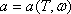(10.7)

It was the first time that “a” was expressed not only as a function of temperature, but also as a function of the shape (sphericity) of the molecules (through w, Pitzer’s acentric factor). As we recall, Pitzer’s acentric factor is a measure of the configuration and sphericity of the molecule. It can also be seen as a measure of the deformity of the molecule.

The Soave-Redlich-Kwong EOS is given by the expression:(10.8a)

Like all cubic equations of state, the SRK EOS is also explicit in pressure. Notice, for example, how the SRK EOS readily becomes:(10.8b)

where,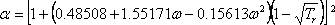(10.8c)

The influence of acentric factor and temperature on the attractive term is introduced now through “a”. What do we do next? We apply the criticality conditions to equation (10.8b). Notice that expression (10.8c) becomes unity at Tr=1, throughout the critical isotherm. We obtain:(10.9a)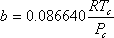(10.9b)

Now we show the cubic form (in compressibility factor) of Soave-Redlich-Kwong EOS. Defining,(10.10a)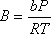, (10.10b)

we are able to obtain:(10.11)

For mixtures, Soave proposed a “little” modification to the mixing rules with which we have dealt with so far by introducing the use of “binary interaction parameters” (kij):;(10.12a)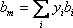(10.12b)

The use of binary interaction parameters (kij) generated a lot of resistance upon their first introduction. This is because there is no analytical, science-based derivation that justifies their existence. Nowadays, they are regarded just as they are, empirical factors used to tune equations of state and make them match experimental data for mixtures. This has become the heuristic justification for their existence: with them, EOS can do a better job of matching experimental data. Heuristicallyspeaking, they are a measure of interaction between a pair of dislike molecules. Based on this “definition,” their value is zero for pairs of molecules that are alike. Actually, this is no more than a mathematical requirement in order for equation (10.12a) to givewhen j=i. The determination of kij is based on experimental data from binary systems; “kij” results from the value that allows the given equation of state (through the expression in 10.12a) to yield the closest match. These values are assumed to be constant (and so are used) when the same two components are part of a more complex multi-component mixture.

## Contributors

• Prof. Michael Adewumi (The Pennsylvania State University). Some or all of the content of this module was taken from Penn State's College of Earth and Mineral Sciences' OER Initiative.